# Elaine Hale Wotao Yin Yin Zhang Computational and

• Slides: 26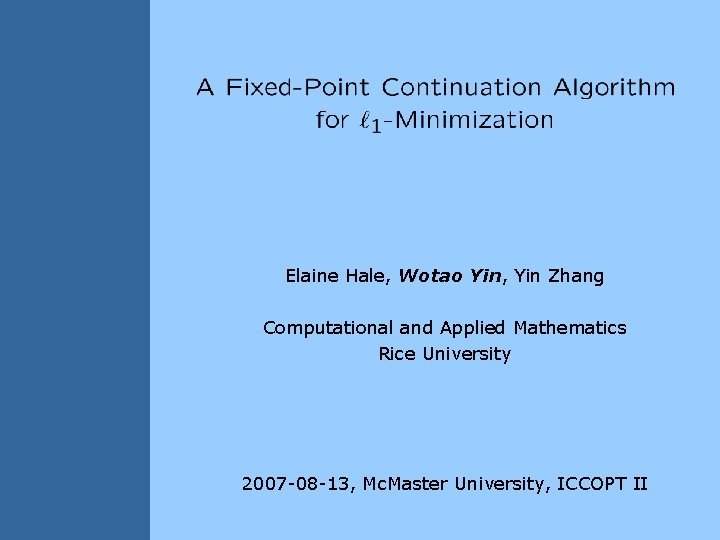Elaine Hale, Wotao Yin, Yin Zhang Computational and Applied Mathematics Rice University 2007 -08 -13, Mc. Master University, ICCOPT IIThe FPC (fixed-point continuation) algorithm A short introduction to Compressed Sensing • An imaging perspective 10 Mega Pixels Scene Picture • Image compression Why do we compress images? 2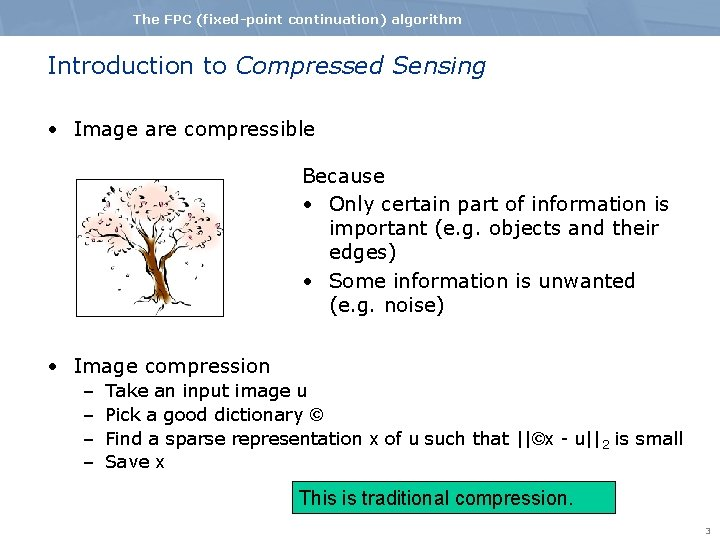The FPC (fixed-point continuation) algorithm Introduction to Compressed Sensing • Image are compressible Because • Only certain part of information is important (e. g. objects and their edges) • Some information is unwanted (e. g. noise) • Image compression – – Take an input image u Pick a good dictionary © Find a sparse representation x of u such that ||©x - u||2 is small Save x This is traditional compression. 3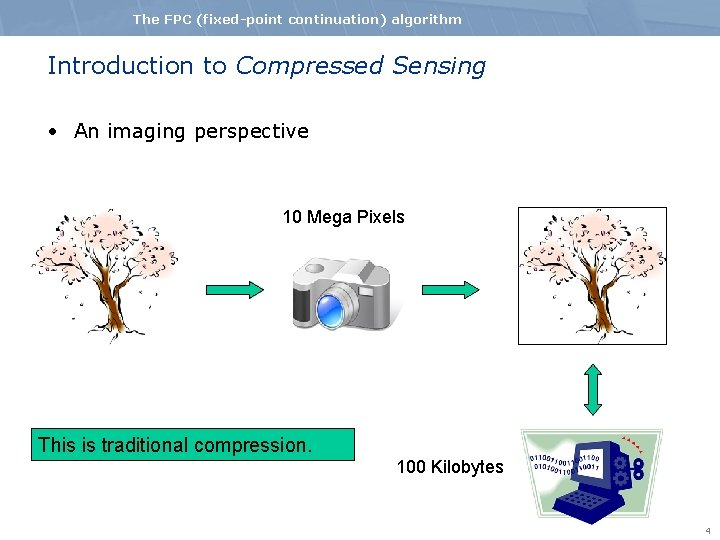The FPC (fixed-point continuation) algorithm Introduction to Compressed Sensing • An imaging perspective 10 Mega Pixels This is traditional compression. 100 Kilobytes 4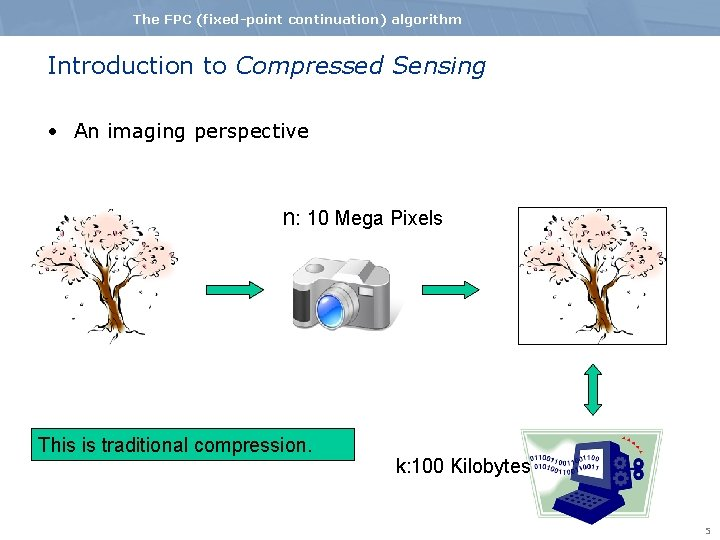The FPC (fixed-point continuation) algorithm Introduction to Compressed Sensing • An imaging perspective n: 10 Mega Pixels This is traditional compression. k: 100 Kilobytes 5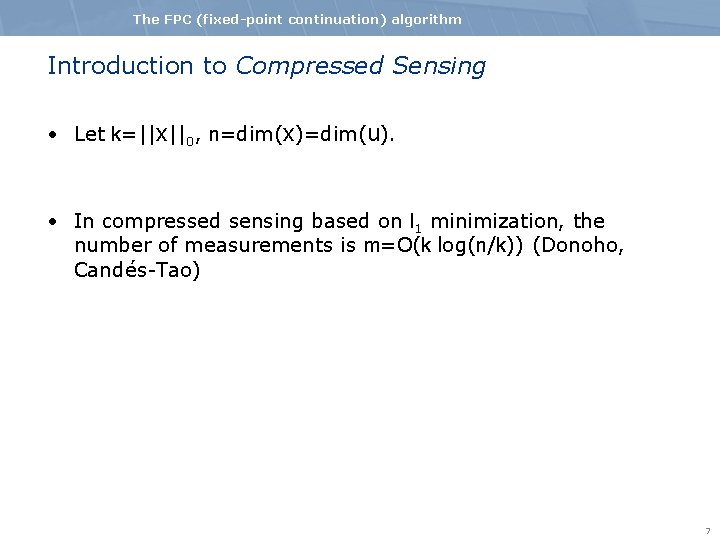The FPC (fixed-point continuation) algorithm Introduction to Compressed Sensing • Let k=||x||0, n=dim(x)=dim(u). • In compressed sensing based on l 1 minimization, the number of measurements is m=O(k log(n/k)) (Donoho, Candés-Tao) 7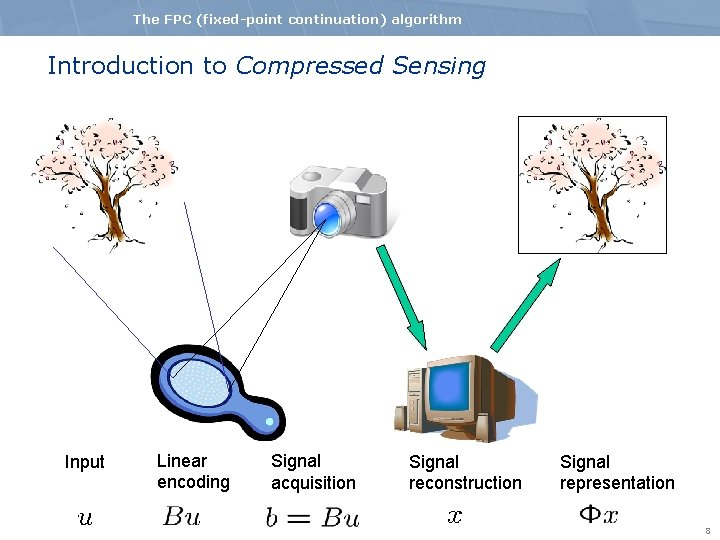The FPC (fixed-point continuation) algorithm Introduction to Compressed Sensing Input Linear encoding Signal acquisition Signal reconstruction Signal representation 8The FPC (fixed-point continuation) algorithm Introduction to Compressed Sensing Input Linear encoding Signal acquisition Signal reconstruction Signal representation 9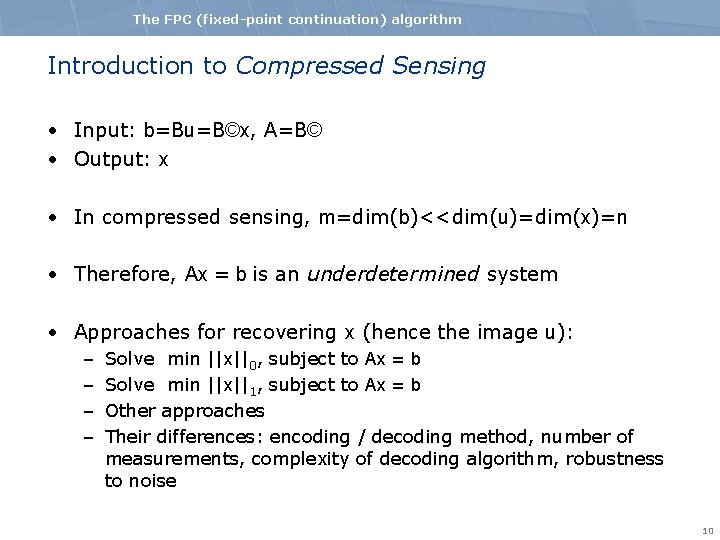The FPC (fixed-point continuation) algorithm Introduction to Compressed Sensing • Input: b=Bu=B©x, A=B© • Output: x • In compressed sensing, m=dim(b)<<dim(u)=dim(x)=n • Therefore, Ax = b is an underdetermined system • Approaches for recovering x (hence the image u): – – Solve min ||x||0, subject to Ax = b Solve min ||x||1, subject to Ax = b Other approaches Their differences: encoding / decoding method, number of measurements, complexity of decoding algorithm, robustness to noise 10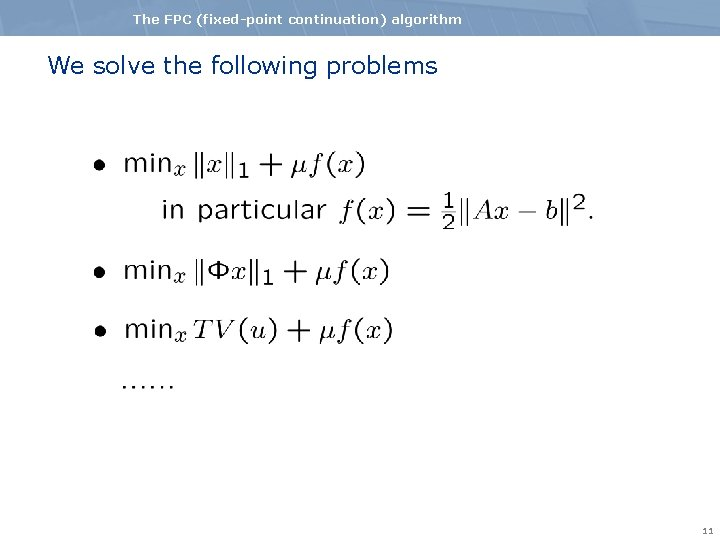The FPC (fixed-point continuation) algorithm We solve the following problems 11The FPC (fixed-point continuation) algorithm Existing approaches • Methods exactly solving the l 1 -problem – – – GPSR (Figueiredo, Nowak, Wright) Iterative Shresholding (Daubechies, Defrise, and De Mol) l 1_ls (Kim, Koh, Lustig, Boyd, Gorinvesky) l 1_magic (Candes, Romberg) Lasso, LARS …… • Methods not solving the l 1 -problem per se – OMP, St. OMP, Random Projections, Sparse Tree Rep. , Belief Propogation, Gradient Pursuits, Sparsify, Randomized Algorithms, Chaining Pursuit, HHS Pursuit. 12The FPC (fixed-point continuation) algorithm Difficulties • Large scales • Completely dense data: A However • Solutions x are expected to be sparse • The matrices A are often fast transforms 13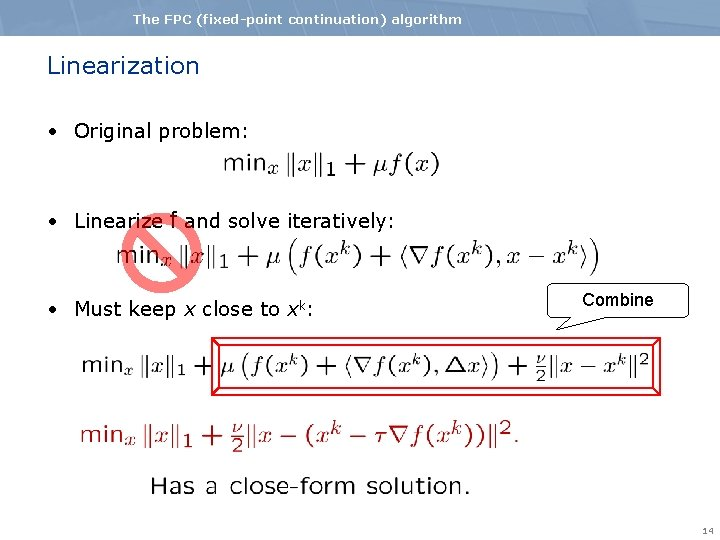The FPC (fixed-point continuation) algorithm Linearization • Original problem: • Linearize f and solve iteratively: • Must keep x close to xk: Combine 14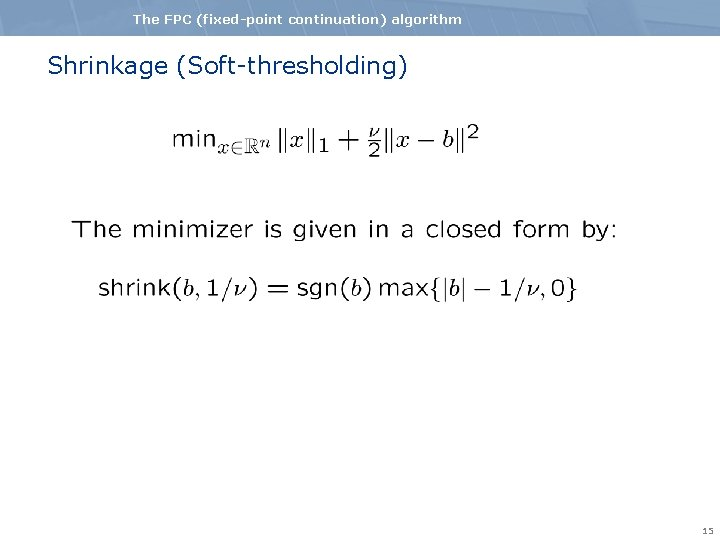The FPC (fixed-point continuation) algorithm Shrinkage (Soft-thresholding) 15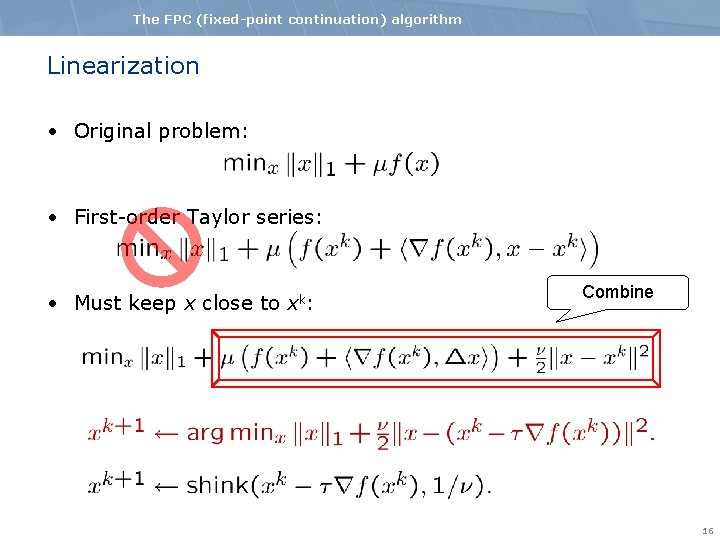The FPC (fixed-point continuation) algorithm Linearization • Original problem: • First-order Taylor series: • Must keep x close to xk: Combine 16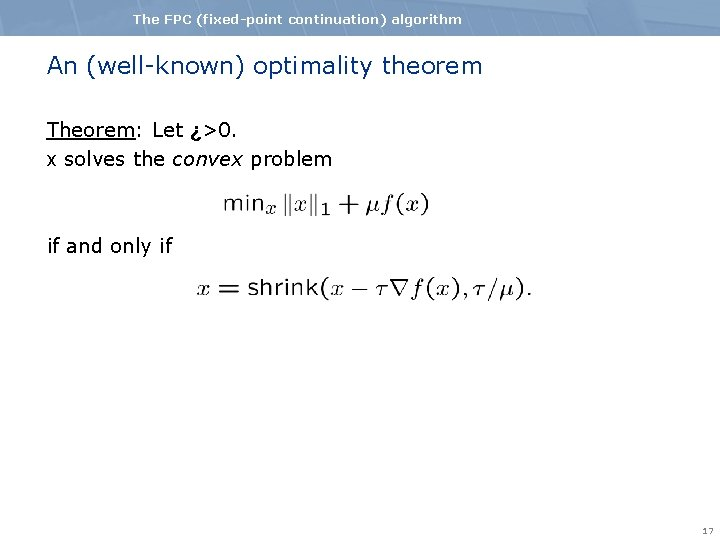The FPC (fixed-point continuation) algorithm An (well-known) optimality theorem Theorem: Let ¿>0. x solves the convex problem if and only if 17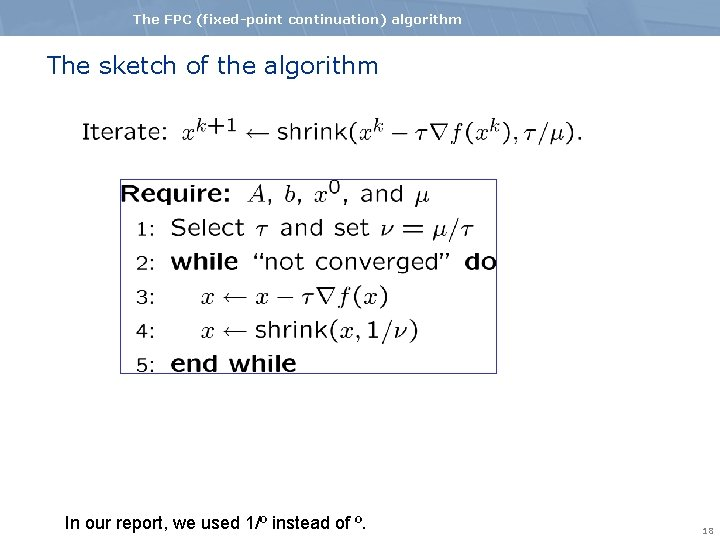The FPC (fixed-point continuation) algorithm The sketch of the algorithm In our report, we used 1/º instead of º. 18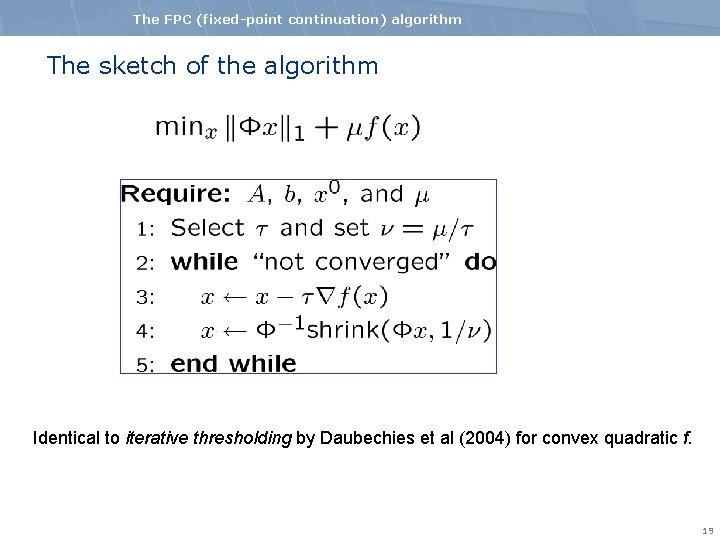The FPC (fixed-point continuation) algorithm The sketch of the algorithm Identical to iterative thresholding by Daubechies et al (2004) for convex quadratic f. 19The FPC (fixed-point continuation) algorithm The sketch of the algorithm 20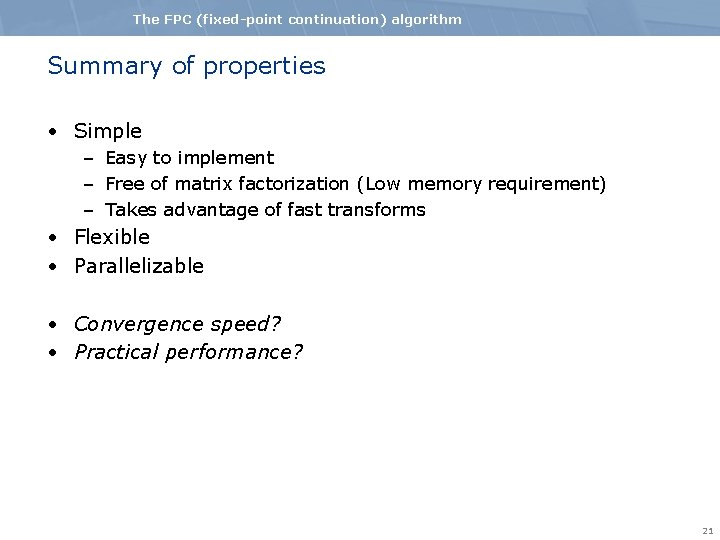The FPC (fixed-point continuation) algorithm Summary of properties • Simple – Easy to implement – Free of matrix factorization (Low memory requirement) – Takes advantage of fast transforms • Flexible • Parallelizable • Convergence speed? • Practical performance? 21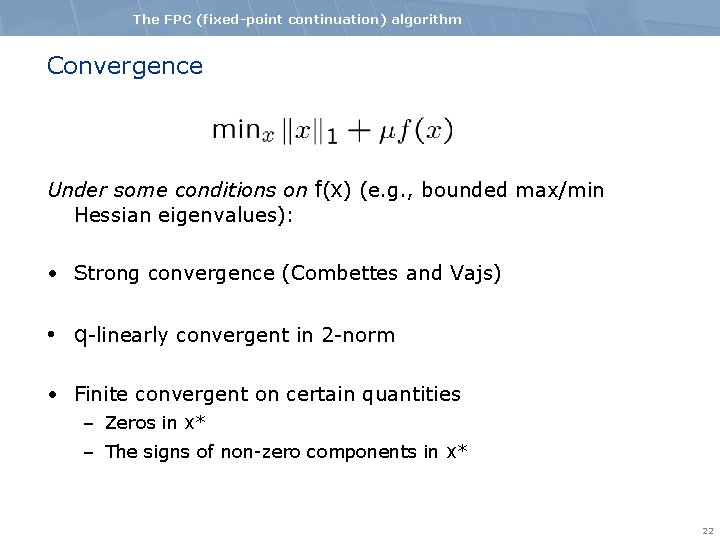The FPC (fixed-point continuation) algorithm Convergence Under some conditions on f(x) (e. g. , bounded max/min Hessian eigenvalues): • Strong convergence (Combettes and Vajs) • q-linearly convergent in 2 -norm • Finite convergent on certain quantities – Zeros in x* – The signs of non-zero components in x* 22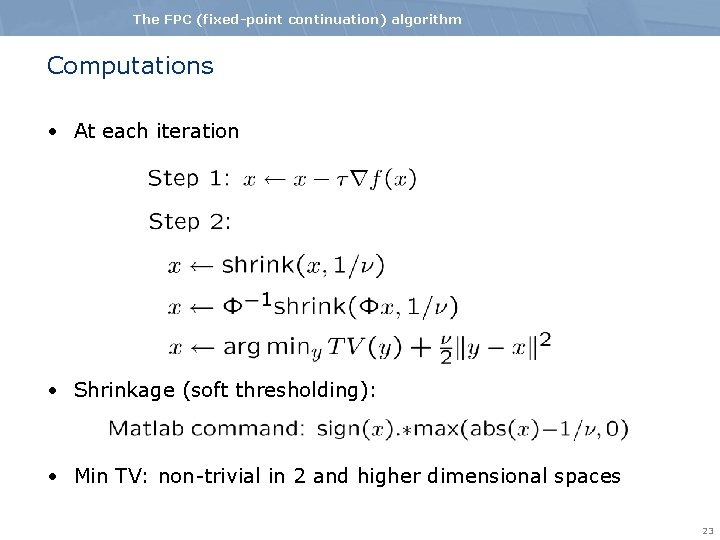The FPC (fixed-point continuation) algorithm Computations • At each iteration • Shrinkage (soft thresholding): • Min TV: non-trivial in 2 and higher dimensional spaces 23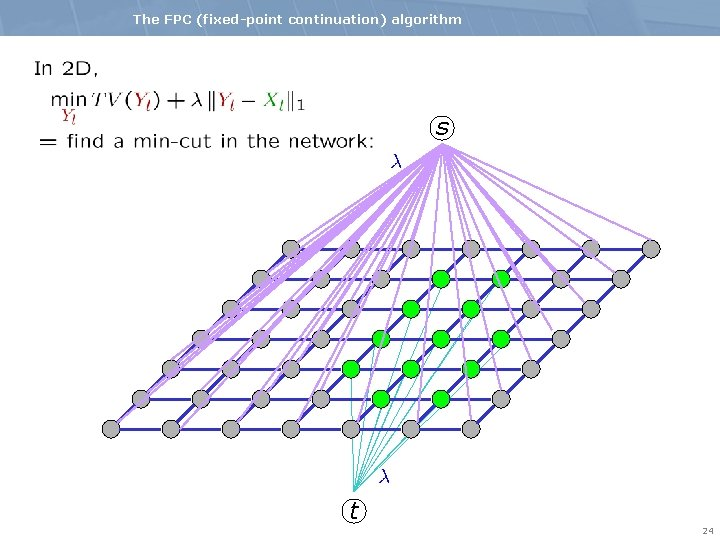The FPC (fixed-point continuation) algorithm s t 24The FPC (fixed-point continuation) algorithm Computations • At each iteration • Shrinkage: • TV minimization: parametric max-flow (see our Poster) 25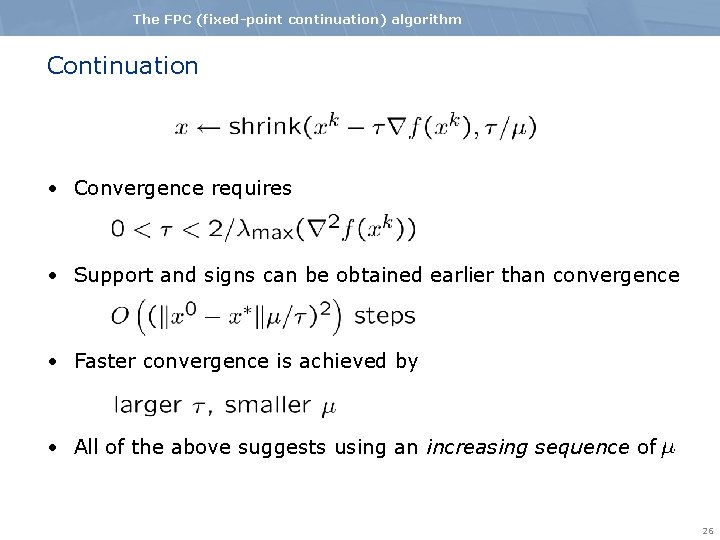The FPC (fixed-point continuation) algorithm Continuation • Convergence requires • Support and signs can be obtained earlier than convergence • Faster convergence is achieved by • All of the above suggests using an increasing sequence of 26The FPC (fixed-point continuation) algorithm Some References • I. Daubechies, M. Defrise, C. De Mol. Iterative Thresholding, CPAM, 2004. • D. Donoho, Y. Tsaig, I. Drori, J. -C. Starck. St. OMP, preprint, 2006 • M. Figueiredo, R. Nowak, S. Wright. GPSR, preprint, 2007. • S. Kim, K. Koh, M. Lustig, S. Boyd, D. Gorinevsky. l 1_ls, preprint, 2007. • E. Candes, J. Romberg. l 1 -magic. 2005 • A report on FPC is available on my homepage. 27